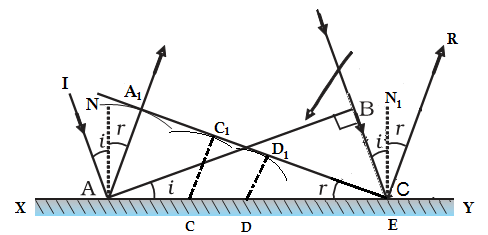# Laws of reflection on the basis of Huygen’s Wave Theory

We can prove the laws of reflection on the basis of Huygens’s wave theory as follows:Light wave reflection on the plane surface

In the given figure, AB is the wavefront incident on a reflecting surface XY with an angle of incidence i as shown in figure. According to Huygen’s principle, every point on AB acts as a source of secondary wavelets. At first, wave incidents at point A and then to points C, D and E. They form a sphere of radii AA1, CC1 and DD1 as shown in figure.

A1E represents the tangential envelop of secondary wavelet in forward direction.

In ΔABE and ΔAA1E,

∠ABE = ∠AA1E = 90°

Side AE = Side AE

AA1 = BE = distance travelled by wave in same time

So, these triangles are congruent.

So, ∠BAE = i and ∠BEA =r

Thus, i = r

Hence, angle of incidence is equal to the angle of reflection. This is the first law of reflection.

Since, the incident wavefront AB, normal and reflected wavefront A1E lies in same plane, it verifies the second law of reflection.

In this way, laws of reflection is verified by Huygens’s principle.

Do you like this article ? If yes then like otherwise dislike : 81

#### 19 Responses to “Laws of reflection on the basis of Huygen’s Wave Theory”

1.Surajkumar. Nishant

Good answer felling happy ,now I can got 5 marks in board exam.

2.ranjan kumar

•Vishant raj

3.If angle BEA=r then according to figure D1DE=r. Confusion.

4.iuioj

great

5.HSEB ko full marks ta haina 45 marks ko question chaiyo vane contact me…..

6.Hey dude this process is totally wrong because you prove triangle ABE and triangle AA¡E is congruent by A.S.S axiom whis is not exist….please fix it.

•himanta

bro its R.H.S axiom its totally right

7.David subu

I’m considering the angle BEA=r..I think it should BEA1= r..

8.David subu

I’m considering the angle BEA=r..I think it should be BEA1= r..

9.Jalesh Kabi

Matter is O. K. But it requires more and clear clarification.

10.Jalesh Kabi

Matter is O. K. But it requires more and clear clarification.

11.sugyan kumar sahu

i want detail notes of laws of reflection and laws of refraction on the basic of Huygen’s wave theory

12.Bhagavan

I like know proof of laws of reflection

13.Asif

14.Saswata Mukherjee

•Saswata Mukherjee

Very very very very bad, worse

15.Rajeev singh

16.